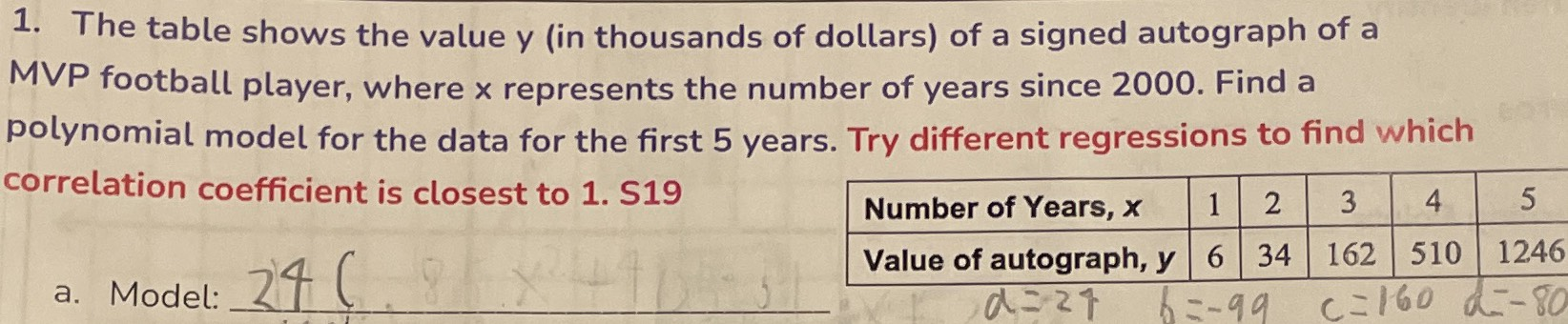### ¿Todavía tienes preguntas de matemáticas?

Pregunte a nuestros tutores expertos
Algebra
Pregunta1. The table shows the value y (in thousands of dollars) of a signed autograph of a MVP football player, where $$x$$ represents the number of years since $$2000$$ . Find a polynomial model for the data for the first $$5$$ years. Try different regressions to find which correlation coefficient is closest to 1. $$519$$

$$y = 24x^{3}- (99*14)x^{2}+ (160*86)x- (80*4)$$